# Fraction calculator

The calculator performs basic and advanced operations with fractions, expressions with fractions combined with integers, decimals, and mixed numbers. It also shows detailed step-by-step information about the fraction calculation procedure. Solve problems with two, three, or more fractions and numbers in one expression.

## Result:

### 3/8 + 1/2 = 7/8 = 0.875

Spelled result in words is seven eighths.

### How do you solve fractions step by step?

1. Add: 3/8 + 1/2 = 3/8 + 1 · 4/2 · 4 = 3/8 + 4/8 = 3 + 4/8 = 7/8
For adding, subtracting, and comparing fractions, it is suitable to adjust both fractions to a common (equal, identical) denominator. The common denominator you can calculate as the least common multiple of both denominators - LCM(8, 2) = 8. In practice, it is enough to find the common denominator (not necessarily the lowest) by multiplying the denominators: 8 × 2 = 16. In the next intermediate step, the fraction result cannot be further simplified by canceling.
In words - three eighths plus one half = seven eighths.

#### Rules for expressions with fractions:

Fractions - use the slash “/” between the numerator and denominator, i.e., for five-hundredths, enter 5/100. If you are using mixed numbers, be sure to leave a single space between the whole and fraction part.
The slash separates the numerator (number above a fraction line) and denominator (number below).

Mixed numerals (mixed fractions or mixed numbers) write as non-zero integer separated by one space and fraction i.e., 1 2/3 (having the same sign). An example of a negative mixed fraction: -5 1/2.
Because slash is both signs for fraction line and division, we recommended use colon (:) as the operator of division fractions i.e., 1/2 : 3.

Decimals (decimal numbers) enter with a decimal point . and they are automatically converted to fractions - i.e. 1.45.

The colon : and slash / is the symbol of division. Can be used to divide mixed numbers 1 2/3 : 4 3/8 or can be used for write complex fractions i.e. 1/2 : 1/3.
An asterisk * or × is the symbol for multiplication.
Plus + is addition, minus sign - is subtraction and ()[] is mathematical parentheses.
The exponentiation/power symbol is ^ - for example: (7/8-4/5)^2 = (7/8-4/5)2

#### Examples:

subtracting fractions: 2/3 - 1/2
multiplying fractions: 7/8 * 3/9
dividing Fractions: 1/2 : 3/4
exponentiation of fraction: 3/5^3
fractional exponents: 16 ^ 1/2
adding fractions and mixed numbers: 8/5 + 6 2/7
dividing integer and fraction: 5 ÷ 1/2
complex fractions: 5/8 : 2 2/3
decimal to fraction: 0.625
Fraction to Decimal: 1/4
Fraction to Percent: 1/8 %
comparing fractions: 1/4 2/3
multiplying a fraction by a whole number: 6 * 3/4
square root of a fraction: sqrt(1/16)
reducing or simplifying the fraction (simplification) - dividing the numerator and denominator of a fraction by the same non-zero number - equivalent fraction: 4/22
expression with brackets: 1/3 * (1/2 - 3 3/8)
compound fraction: 3/4 of 5/7
fractions multiple: 2/3 of 3/5
divide to find the quotient: 3/5 ÷ 2/3

The calculator follows well-known rules for order of operations. The most common mnemonics for remembering this order of operations are:
PEMDAS - Parentheses, Exponents, Multiplication, Division, Addition, Subtraction.
BEDMAS - Brackets, Exponents, Division, Multiplication, Addition, Subtraction
BODMAS - Brackets, Of or Order, Division, Multiplication, Addition, Subtraction.
GEMDAS - Grouping Symbols - brackets (){}, Exponents, Multiplication, Division, Addition, Subtraction.
Be careful, always do multiplication and division before addition and subtraction. Some operators (+ and -) and (* and /) has the same priority and then must evaluate from left to right.

## Fractions in word problems:

• SomeoneSomeone ate 1/10 of a cake, leaving only 9/10. If you eat 2/3 of the cake that is left, how much of a whole cake will you have eaten?
• A baker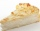A baker has 5 1/4 pies in her shop. She cut the pies in pieces that are each 1/8 of a whole pie. How many pieces of pie does she have?
• Cakes1/3 poppy cake, 1/3 apple, 15 pieces of cheese. How many are totally cakes?
• Bricklayers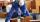8 bricklayers build a house for 630 days. How many bricklayers have to add after 150 days to complete the whole building in (next) 320 days?2 and 1/8th plus 1 and 1/3rd =
• Unknown number 23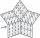Find 2/3 of unknown number, which is two thirds of the 99.
• Tailor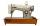Tailor bought 2 3/4 meters of textile and paid 638 CZK. Determine the price per 1 m of the textile.Mom bake cookies. Rolo took 2/9 of all cookies, Michal 3/9. How many cookies ate Rolo if Michal had 9.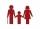I'm a primary school pupil. I attended the parents' exercises with children 1/4 of my age, 1/3 for drawing, and 1/6 for flute. For the first three years of my life, I had no ring, and I never went to two rings simultaneously. How old am I?Ann ate a third of a pizza and then another quater. Total part of pizza eaten by Ann and how much pizza is left?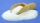Heather has 2 cups of powdered sugar. She sprinkles 3/5 of the sugar onto a plate of brownies and sprinkles the rest into a plate of lemon cookies. How much sugar does Heather sprinkle on the brownies? How much sugar does Heather sprinkle on the lemon coo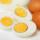A dozen eggs cost 80 cents . how much will 3½ dozen eggs cost?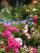On the large rosary was a third white, half red, yellow quarter and six pink. How many roses was in the rosary?